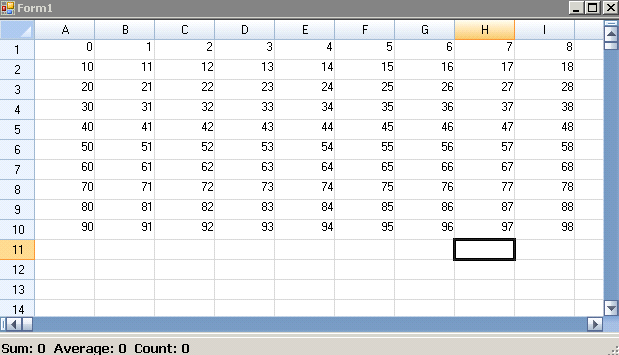﻿ Summarizing Selected Cells in Spread# Summarizing Selected Cells in Spread

It won't be an exaggeration by declaring that Spread is the world's #1 selling spreadsheet component for Microsoft Visual Studio. There are many factors that contributes to this very special distinction : Data Visualization, Code-free Development and Extensive Customization Options are just to name a few. However, the biggest and most explicable factor is its compatibility with Microsoft Excel. The fact that installing Microsoft Excel is not necessary for Spread, makes it even more overwhelming. Spread has proven well in providing the industry's best native Excel support. You can import data from and export data (and formatting) to Excel, including XLS, XLSX, CSV, and TXT files. Apart from compatibility with Microsoft Excel, Spread has been created to have the flexibility to be modified or customized to include a wide range of features. One such feature which we found is a Microsoft Excel behaviour. You must have seen the sum, count and average of numbers of the selected cells in the status bar of an Excel file.This feature although small is very effective in cases where quick calculation for the grid's columns or rows are required. This can be accomplished in Spread using a very simple routine: 1. Use SelectionChanging and CellClick events of Spread. 2. Retrieve the selected cell range. 3. Parse these selected cells to calculate the sum, average and count.

``````
int _iRowCount;
int _iColumnCount;
Double sum = 0;
int count = 0, avgCount = 0;
if (e.Range.Column == -1){
for (int i = e.Range.Row; i &lt; e.Range.Row + e.Range.RowCount; i++)
{
try{
sum = sum + Double.Parse(fpSpread1.ActiveSheet.Cells[i, j].Text);
avgCount++;
count++;
}
}
catch{
count++;
}
}
}
else if (e.Range.Row == -1)
{
{
for (int j = e.Range.Column; j &lt; e.Range.Column + e.Range.ColumnCount; j++)
try{
sum = sum + Double.Parse(fpSpread1.ActiveSheet.Cells[i, j].Text);
avgCount++;
count++;
}
}
catch{
count++;
}
}
}
else
{
for (int i = e.Range.Row; i &lt; e.Range.Row + e.Range.RowCount; i++)
{
for (int j = e.Range.Column; j &lt; e.Range.Column + e.Range.ColumnCount; j++)
try{
sum = sum + Double.Parse(fpSpread1.ActiveSheet.Cells[i, j].Text);
avgCount++;
count++;
}
}
catch{ if (fpSpread1.ActiveSheet.Cells[i, j].Text != string.Empty)
count++;
}
}
}
LblSum.Text = &quot;Sum: &quot; + sum;
if (avgCount &gt; 0)
LblAVG.Text = &quot;Average: &quot; + (sum / (Double)avgCount);
else
LblAVG.Text = &quot;Average: 0&quot;;
LblCNT.Text = &quot;Count: &quot; + count;
}

Double sum = 0;
int count = 0, avgCount = 0;
{
{
try{
sum = sum + Double.Parse(fpSpread1.ActiveSheet.Cells[e.Row, i].Text);
avgCount++;
count++;
}
}
catch{
count++;
}
}
}
{
try{
sum = sum + Double.Parse(fpSpread1.ActiveSheet.Cells[i, e.Column].Text);
avgCount++;
count++;
}}

catch{
count++;
}
}
}
LblSum.Text = &quot;Sum: &quot; + sum;
if (avgCount &gt; 0)
LblAVG.Text = &quot;Average: &quot; + (sum / (Double)avgCount);
else
LblAVG.Text = &quot;Average: 0&quot;;
LblCNT.Text = &quot;Count: &quot; + count;
}

``````

Thus, you can see the sum of selected cells in the status bar of the Form. Stay tuned in on ComponentOne for more Spread tips :) Download C# Sample Download VB SampleGrapeCity

GrapeCity Developer Tools